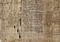# Ancient Egyptians: The Original Computer Scientists?

Renowned by the Greeks as masters of geometry, very little remains of complex Egyptian mathematics. Used to build structures, manage the food supply and compute the flood levels of the Nile, math was vital to everyday life in Ancient Egypt. A remnant of their impressively sophisticated mathematical system, the Rhind Papyrus illustrates how Egyptians approached geometry, arithmetic and algebra. Architecture marvels, the pyramids were built using equations showcased in the Rhind Papyrus. Demonstrations of multiplication and division, using a binary system, are also present in the Rhind Papyrus.A portion of the Rhind Papyrus hanging in the British Museum.

# Ancient Egyptian Calculations

Using a method of doubling and halving, Ancient Egyptians based their multiplication and division on the same binary system that is used today in modern computers. Like today’s computer programmers, Ancient Egyptians knew that all numbers can be expressed as a power of two. This is the same approach rapidly executed by computers.

# Example of Binary Multiplication

When performing multiplication with a binary system, the first number is determined in binary. The second number is doubled and then added together. In the example above, 4 x 64 is equal to 256 in decimal form.

# Example of Binary Division

When performing division with a binary system, the second number is doubled and aligned with the powers of two. The highest doubling less than the number being divided is subtracted and a 1 is noted. In the above example, the closest doubled number under 120 is 80, then 40, with no remainder. The matching levels ultimately amount to the binary number 00110, which is 12 in decimal form. Therefore, 120/10 is equal to 12.

# Modern Computers

Requiring at least two characters to function, modern information systems use the binary system. The smallest set available, computers use the binary system to indicate ‘on’ or ‘off’, voltage or no voltage. Breaking down and processing information in zeros and ones, modern computers mirror the way Ancient Egyptians manipulated their number system.

Making use of a binary number system over 3,000 years before Gottfried Wilhelm Leibniz introduced it to the Western world, Ancient Egyptians pioneered the mathematical approach that is now the basis for modern computer systems. Known for their advanced mathematics, the Ancient Egyptians also developed a variety of concepts that we still use today, including board games, clocks and toothpaste. Leaving behind one of the most remarkable creations of classical antiquity, the pyramids continue to showcase the inventive prowess of Ancient Egypt.

Written by